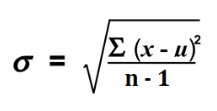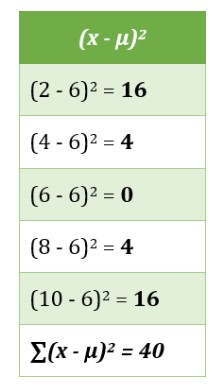# Coefficient of Variation Calculator

To find the coefficient of variation enter comma-separated values and click the calculate button using coefficient of variation calculator

Formula:
CV = σμ CV = s
Sample Population

Give Us Feedback

## Coefficient of Variation Calculator

Coefficient of variation calculator finds the coefficient of variation by taking the range of values as input. If you are wondering how to calculate the coefficient of variation, then, stop being too curious, because we are going to elaborate on all that in the next sections.

## What is the coefficient of variation?

In statistics, the coefficient of variation is the ratio of standard deviation to mean. It is a measure of relative variability. It is also known as relative standard deviation. The higher coefficient of variation (CV) depicts the greater dispersion around the mean.

## Coefficient of variation formula

The formula for CV can be expressed as:

CV = σ/μ

Where,

• CV = Coefficient of variation,
• σ = Standard deviation, and
• μ = Sample mean.

## How to find the coefficient of variation?

Here is an example to find CV.

Example:

Find the coefficient of variation for sample 2, 4, 6, 8, 10.

Solution:

Step 1: Calculate the sample mean.

μ = ∑x/n = (2+4+6+8+10)/5

μ = 6

Step 2: Calculate the standard deviation using the below formula.σ =√ (40/ (5-1))

σ = 3.16

Step 3: Place the values in the CV formula.

CV = σ/μ = 3.16/6

CV = 0.5271

Use the CV calculator to save time if you don’t like to play with formulas and equations.

## References

### Math Tools